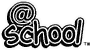Converting Units of Length -1 Classroom Keystage 2-Maths - Measures - Length, Mass and Capacity

[ To answer the questions please circle the correct answer or fill in the blank spaces ]

 Converting Units of Length - 1There are 1000 metres (m) in every kilometre (km)There are 100 centimetres (cm) in every metre(m)There are 10 millimetres (mm) in every centimetre (cm)TYPE in the correct answer for each of these conversions.1) 1000m [_] km          2) 1500m [___] km          3) 2800m [___] km4) 1km [____] m          5) 1.2km [____] m          6) 0.5km [___] m7) 1m [___] cm          8) 1.25m [___] cm          9) 2.78m [___] cm

© Copyright Pedegog Ltd All rights reserved - www.atschool.co.uk